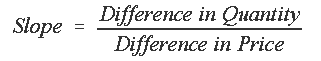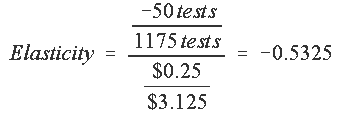## Elasticity

Elasticity of demand is a measure of the responsiveness of the demanded quantity to price changes.

The elasticity of demand for something is:For instance, If price goes up 1% and as a result sales fall 2%, the elasticity is -2%/1%=-2.
Elasticity, as a measure of responsiveness to price change, is an alternative to the slope, which would beExample of calculation (from Phil Jacobs' book):
 Health Dept. charge per shot Shots done per month \$3 1200 \$3.25 1150

Slope of demand for tests is
-50 tests / \$0.25 = -200 tests per \$ of price change.I used 1175 and \$3.125 in the elasticity formula. These are averages of the before and after quantity and price levels.  1175 = (1150+1200)/2.  \$3.125 = (\$3.00 + \$3.25)/2.  The elasticity calculated this way is called the arc elasticity.

Notice how the slope has units (tests per dollar), but the elasticity does not.  The elasticity has no units because the numerator is tests/tests and the denominator is \$/\$.  All the units cancel out, leaving a unitless number.  This means you get the same answer regardless of what units you use.  This lets you compare elasticities of totally different products, like medical care, petroleum, and DVD rentals.

One interpretation of elasticity of demand:
It's the percentage change in quantity demanded that comes from a 1% change in the price.

Here's another way to interpret elasticity of demand:
Suppose you raise the price of something you're selling.  Will you make more money?  The answer depends on the elasticity of demand.

 Elasticity (absolute value, ignoring the minus sign) If price goes up, Demand is categorized as: > 1 spending goes down. Elastic = 1 spending stays the same. Unitary elasticity < 1 spending goes up. Inelastic

What are examples of products or services whose demand is elastic?  Inelastic?

Hot tip:  If you want to impress economists, say:  "That depends on the elasticity, doesn't it?"

The small elasticities observed for medical care demand imply that higher med care prices will cause people to cut back some, but they will not much, so the total amount spent on care will go up.

Elasticity can be used for other things besides quantities and prices.

For example, you can talk about the income elasticity of spending on health care. An elasticity of 1 would mean that as income rises by 1%, health care spending rises by 1%.

For another example, you could talk about the elasticity of health status with respect to medical care expenditure. This would be the percentage difference in health status divided by the percentage difference in medical care expenditure.

The advantage of elasticity over slope is that, as mentined,